# Logic Gates A+ A  A-

BOOLEAN CONSTANTS AND VARIABLES
Boolean algebra differs in a major way from ordinary algebra because Boolean constants and variables are allowed to have only two possible values, 0 or 1. A Boolean variable is a quantity that may, at different times, be equal
to either 0 or 1. Boolean variables are often used to represent the voltage level present on a wire or at the input/output terminals of a circuit. For example, in a certain digital system, the Boolean value of 0 might be assigned
to any voltage in the range from 0 to 0.8 V, while the Boolean value of 1 might be assigned to any voltage in the range 2 to 5 V.

Thus, Boolean 0 and 1 do not represent actual numbers but instead represent the state of a voltage variable, or what is called its logic level.

As we said in the introduction, Boolean algebra is a means for expressing the relationship between a logic circuit’s inputs and outputs. The inputs are considered logic variables whose logic levels at any time determine the output levels

Because only two values are possible, Boolean algebra is relatively easy to work with compared with ordinary algebra. In Boolean algebra, there are no fractions, decimals, negative numbers, square roots, cube roots, logarithms, imaginary numbers, and so on. In fact, in Boolean algebra there are only three basic operations: OR, AND, and NOT.
These basic operations are called logic operations. Digital circuits called logic gates can be constructed from diodes, transistors, and resistors connected so that the circuit output is the result of a basic logic operation (OR, AND, NOT) performed on the inputs. We will be using Boolean algebra first to describe and analyze these basic logic gates, then later to analyze and design combinations of logic gates connected as logic circuits.

TRUTH TABLES
A truth table is a means for describing how a logic circuit’s output depends on the logic levels present at the circuit’s inputs

OR OPERATION WITH OR GATES
The OR operation is the first of the three basic Boolean operations to be learned.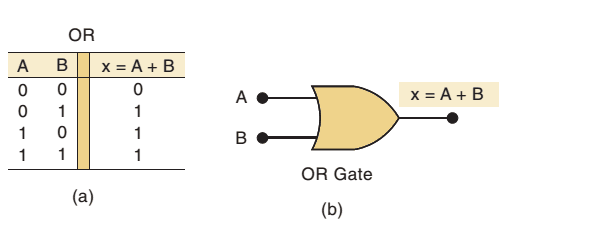The Boolean expression for the OR operation is  x = A + B

OR Gate
In digital circuitry, an OR gate* is a circuit that has two or more inputs and whose output is equal to the OR combination of the inputs.

Summary of the OR Operation
The important points to remember concerning the OR operation and OR gates are:

•  The OR operation produces a result (output) of 1 whenever any input is a 1. Otherwise the output is 0.
•  An OR gate is a logic circuit that performs an OR operation on the circuit’s inputs.
•  The expression is read as “ x = A + B x equals A OR B.”

AND OPERATION WITH AND GATES
The AND operation is the second basic Boolean operation. As an example of the use of AND logic,
The Boolean expression for the AND operation is  x = A . B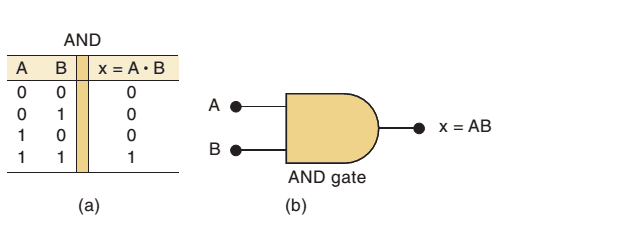Note the difference between the symbols for the AND gate and the OR gate. Whenever you see the AND symbol on a logic-circuit diagram, it tells you that the output will go HIGH only when all inputs are HIGH. Whenever you
see the OR symbol, it means that the output will go HIGH when any input is HIGH.

Summary of the AND Operation

• The AND operation is performed the same as ordinary multiplication of 1s and 0s.
• An AND gate is a logic circuit that performs the AND operation on the circuit’s inputs.
• An AND gate output will be 1 only for the case when all inputs are 1; for all other cases, the output will be 0.
• The expression is read as “ x = AB x equals A AND B.”

NOT OPERATION
The NOT operation is unlike the OR and AND operations because it can be performed on a single input variable. The NOT operation is also referred to as inversion or complementation,NOT Circuit (INVERTER)
circuit always has only a single input, and its output logic level is always opposite to the logic level of this input.
It inverts (complements) the input signal at all points on the waveform so that whenever the input  0, output  1, and vice versa.

Summary of Boolean Operations
The rules for the OR,AND, and NOT operations may be summarized as follows: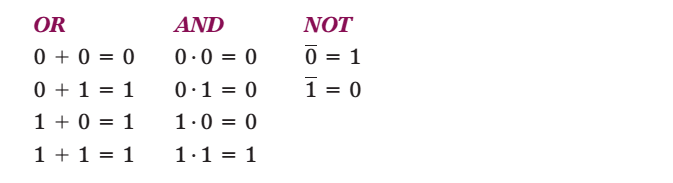NOR Gate
The symbol for a two-input NOR gate is shown in Figure. It is the same as the OR gate symbol except that it has a small circle on the output. The small circle represents the inversion operation. Thus, the NOR gate operates like an OR gate followed by an INVERTER, so that the circuits in Figure are equivalent, and the output expression for the NOR gate is.The truth table in Figure shows that the NOR gate output is the exact inverse of the OR gate output for all possible input conditions. An OR gate output goes HIGH when any input is HIGH; the NOR gate output goes LOW when any input is HIGH. This same operation can be extended to NOR gates with more than two inputs.

NAND Gate
The symbol for a two-input NAND gate is shown in Figure below. It is the same as the AND gate symbol except for the small circle on its output. Once again, this small circle denotes the inversion operation. Thus, the NAND operates like an AND gate followed by an INVERTER, so that the circuits of Figure below (a) and (b) are equivalent, and the output expression for the NAND gate is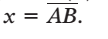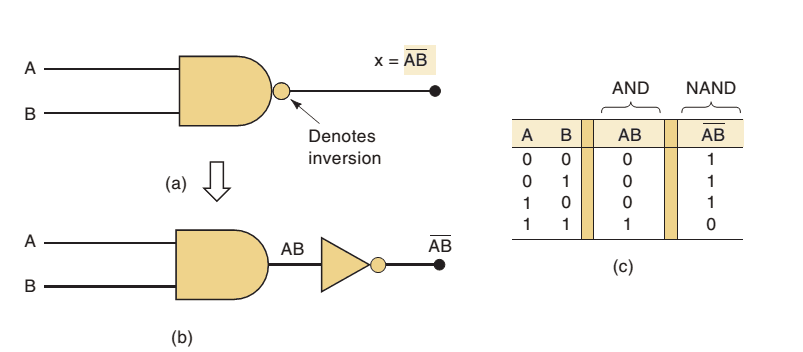The truth table in Figure above shows that the NAND gate output is the exact inverse of the AND gate for all possible input conditions. The AND output goes HIGH only when all inputs are HIGH, while the NAND output goes LOW only when all inputs are HIGH. This same characteristic is true of NAND gates having more than two inputs.